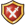Free

Template documentation (for the above template, sometimes hidden or invisible)[view]  [history] [refresh]

Contents

==Description==

This template is used to display values of many kinds with an appropriate icon.

Syntax

Type {{Value|type=|Value=|Percent=|size=}} somewhere.

Examples

Example uses
Code Output Code Output
{{Value}}
Free
{{Value
|type = xp
}}{{Value
|type = xp
|Value = 10
}}10
{{Value
|type = xp
|Percent = +10
}}+10%
{{Value
|type = time
}}{{Value
|type = time
|Value = 1d23hr59m59s
}}1d23hr59m59s
{{Value
|type = time
|Percent = +10
}}+10%
{{Value
|type = power
}}{{Value
|type = power
|Value = 10
}}10
{{Value
|type = power
|Percent = +10
}}+10%
{{Value
|type = heart
}}{{Value
|type = heart
|Value = 10
}}10
{{Value
|type = heart
|Percent = +10
}}+10%
{{Value
|type = chest
}}{{Value
|type = chest
|Value = 10
}}10
{{Value
|type = chest
|Percent = +10
}}+10%
{{Value
|type = gem
}}{{Value
|type = gem
|Value = 10
}}10
{{Value
|type = gem
|Percent = +10
}}+10%
{{Value
|type = gold
}}{{Value
|type = gold
|Value = 10
}}10
{{Value
|type = gold
|Percent = +10
}}+10%

{{Value
|type = fragment
}}{{Value
|type = fragment
|Value = 10
}}10
{{Value
|type = fragment
|Percent = +10
}}+10%
The above text is transcluded from Template:Value/doc; you can change it or view its history. (How does this work?)
If you're done making changes, please refresh the text. (Why?)
You can experiment in this template's sandbox (create | mirror) and testcases (create) pages.